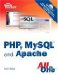# Some Array-Related Functions

 More than 70 array-related functions are built into PHP, which you can read about in detail at http://www.php.net/array. Some of the more common (and useful) functions are explained in this section. count() and sizeof() Each of these functions counts the number of elements in an array. Given the following array`\$colors = array("blue", "black", "red", "green");`both count(\$colors); and sizeof(\$colors); return a value of 4.each() and list() These functions usually appear together, in the context of stepping through an array and returning its keys and values. You saw an example of this previously, where we stepped through the \$c array and printed its contents.foreach() This function is also used to step through an array, assigning the value of an element to a given variable, as you saw in the previous section.reset() This function rewinds the pointer to the beginning of an array, as in this example:`reset(\$character);`This function is useful when you are performing multiple manipulations on an array, such as sorting, extracting values, and so forth.array_push() This function adds one or more elements to the end of an existing array, as in this example:`array_push(\$existingArray, "element 1", "element 2", "element 3");`array_pop() This function removes (and returns) the last element of an existing array, as in this example:`\$last_element = array_pop(\$existingArray);`array_unshift() This function adds one or more elements to the beginning of an existing array, as in this example:`array_unshift(\$existingArray, "element 1", "element 2", "element 3");`array_shift() This function removes (and returns) the first element of an existing array, as in this example, where the value of the element in the first position of \$existingArray is assigned to the variable \$first_element:`\$first_element = array_shift(\$existingArray);`array_merge() This function combines two or more existing arrays, as in this example:`\$newArray = array_merge(\$array1, \$array2);`array_keys() This function returns an array containing all the key names within a given array, as in this example:`\$keysArray = array_keys(\$existingArray);`array_values() This function returns an array containing all the values within a given array, as in this example:`\$valuesArray = array_values(\$existingArray);`shuffle() This function randomizes the elements of a given array. The syntax of this function is simply as follows:`shuffle(\$existingArray);` This brief rundown of array-related functions only scratches the surface of using arrays. However, arrays and array-related functions are used in the code examples throughout this book so you will get your fill soon enough. If you don't, there's always the array section of the PHP Manual at http://www.php.net/array, which discusses all array-related functions in great detail, including more than ten different methods for sorting your arrays.Sams Teach Yourself PHP, MySQL and Apache All in One (3rd Edition)
ISBN: 0672328739
EAN: 2147483647
Year: 2004
Pages: 327